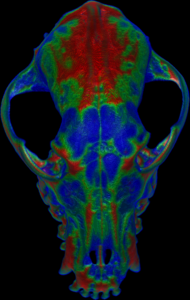## Whole-object measurement (ImageXd)

 scalar.load “test.nii.gz” scalar.fullscan false data := scalar.measure.mean 16 scalar.use data scalar.save “mean.nii.gz”scalar.load “test.nii.gz” scalar.fullscan false data := scalar.measure.sd 16 scalar.use data scalar.save “sd.nii.gz”scalar.load “test.nii.gz” scalar.fullscan data := scalar.measure.thickness 16 scalar.use data scalar.save “thickness.nii.gz”